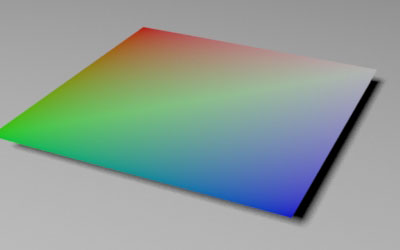### RIBIntroduction to Primvars

#### Introduction

In the RenderMan terminology an item of data that is "bound" to a geometric primitive is called a "primitive variable" or "primvar" for short. The values of a primvar are intended to effect the way a geometric primitive is shaded. This tutorial provides a brief overview of primvars, how they are specified in a rib file and how they are declared in an OSL shader.

Figure 1 shows a polygon that has been shaded using the PxrDisney shader.

```    Bxdf "PxrDisney" "PxrDisney1" "color baseColor" [1 1 0]
Polygon "P" [-0.5 0 -0.5  -0.5 0 0.5  0.5 0 0.5  0.5 0 -0.5]
```Figure 1

In the next snippet of rib, the polygon has been given a primvar named "cs". Note PxrDisney is receiving values from the OSL shader named use_cs - listing 1.

```    Pattern "PxrOSL" "use_cs1" "string shader" "use_cs"
Bxdf "PxrDisney" "PxrDisney1"
"reference color baseColor" ["use_cs1:resultRGB"]
Polygon "P" [-0.5 0 -0.5  -0.5 0 0.5  0.5 0 0.5  0.5 0 -0.5]
"varying color cs" [1 0 0   0 1 0   0 0 1   1 1 1]```

Because the polygon has 4 vertices, the primvar can bind 4 rgb values - one for each vertex. The OSL shader uses "cs" color values that have been smoothly interpolated by PRMan across the surface of the polygon.Figure 2

Listing 1 (use_cs.osl)

 ```shader use_cs(color cs = 1 [[int lockgeom = 0]], output color resultRGB = 0) { resultRGB = cs; }```

In the next snippet of rib an OSL shader called "hole" (listing 2) sets the size of a circular patch of zero "presence" (figure 3).

```    Pattern "PxrOSL" "hole1" "string shader" "hole"
"float blur" 0
Bxdf "PxrDisney" "PxrDisney1"
"reference float presence" ["hole1:resultF"]
"float specular" [0.0]```Figure 3

Listing 2 (hole.osl)

 ```shader hole(float radius = 0.5, float blur = 0, output float resultF = 0) { point p = transform("object", P); point origin = point(0,0,0); float dist = distance(p, origin); resultF = smoothstep(radius - blur, radius + blur, dist); }```

Alternatively, the radius could be controlled by a primvar in which case the OSL shader needs to be modified - listing 3.

```    Pattern "PxrOSL" "hole1" "string shader" "hole"
Bxdf "PxrDisney" "PxrDisney1"
"reference float presence" ["hole1:resultF"]
"float specular" [0.0]
Polygon "P" [-0.5 0 -0.5  -0.5 0 0.5  0.5 0 0.5  0.5 0 -0.5]
```

" Application Note #22 - Class Specifiers for Primitive Variables".

#### OSL Parameter Declaration

The code for a version of the "hole" shader that uses a primvar is given in listing 3. Note the "radius" parameter is tagged as a primvar,

`    float radius = 0.5 [[int lockgeom = 0]]`

Listing 3 (hole.osl)

 ```shader hole(float radius = 0.5 [[int lockgeom = 0]], float blur = 0, output float resultF = 0) { point p = transform("object", P); point origin = point(0,0,0); float dist = distance(p, origin); resultF = smoothstep(radius - blur, radius + blur, dist); }```

The advantage of using a primvar is that several objects can be effected a single shader - figure 4. The rib file used to render the image can be viewed here.Figure 4